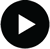## Heat Capacity### Heat, Temperature and Heat CapacityVideo tutorial
Heat and temperature are not the same thing.

It takes more heat to change the temperature of a full saucepan of water than it does to change the temoerature of an empty pan by the same amount. Heat flows from hot to cold objects: it is the transfer of energy due to a temperature difference. "Cold" does not flow from cold objects to hot objects!

The temperature change brought about by supplying an amount of heat depends on:
• the substance being heated up (it takes more heat to warm up the water inside the saucepan than the metal of the saucepan itself
• the amount of the substance (it takes more heat to warm up a full saucepan than a half empty one)
Copper has a low heat capacity meaning that a small amount of heat produces a large temperature change. Water has a high heat capacity meaning that a large amount of heat is required to warm it up.

We use two common measures of amount of stuff in science: mass and number of moles. Depending on which we are using, there are therefore two different heat capacity values for each substance:
• If the amount of material is known in grams, we use the specific heat capacity. This has the symbol c and units J g-1 K-1.
• If the amount of material is known in moles, we use the molar heat capacity. This has the symbol C and units J mol-1 K-1.
The heat, q is thus related to the temperature change, ΔT, by:
For a mass m: For a number of moles n: q = m × c × ΔT q = n × C × ΔT ΔT = q / (m × c) ΔT = q / (n × C)
Points to note:
• ΔT is the temperature difference between the initial temperature, Ti, and the final temperature, Tf such that:
ΔT = ΔTf - ΔTi
• If the temperature increases, Tf > Ti and so ΔT > 0 and so q > 0. Increasing the heat, increases the temperature and vice versa.
• If the temperature decreases, Tf < Ti and so ΔT < 0 and so q < 0. Decreasing the heat, decreases the temperature and vice versa.
• If Tf and Ti are given in °C, there is no need to convert each to Kelvin as the difference between them is the same on the centigrade and Kelvin scales.
• Whenever you calculate a property from a measurement, the signficant figures must be handled correctly, read the iChem online tutorial (identical to the E9 week 4 pre-laboratory information).